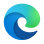Version 1.0, Genesis, is now available.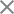# Lenses and Imaging

### Introduction

Lenses are devices made from glass or any other transparent material. The most basic lenses have surfaces with spherical curvature. Lenses got their names from the Latin lēns, which is the Latin name for lentil because a double-convex lens is lentil-shaped. Lenses are used in optical systems to focus light, form an image, or collimate beams. The power of a lens is described by the distance from the lens to the focal point and is denoted as the lens’s focal length.

### Lensmaker's equation

Lenses are usually made from two spherical or plane facets from a transparent material. The schematic of a convex-convex lens is illustrated in Fig. 1, together with its parameters and its influence on a collimated beam showing the focal point.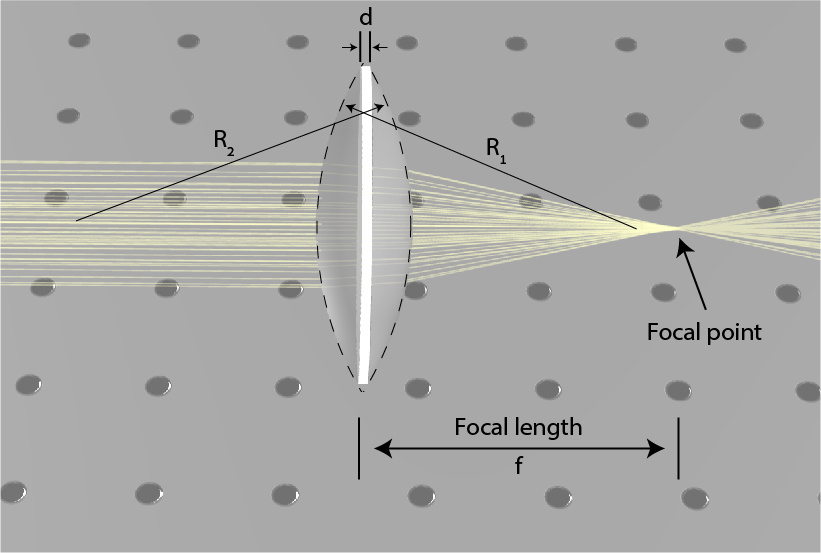Fig. 1. Schematics of a focusing lens, showing the focal length of the lens as the distance to the focal point, and all the lens parameters.
The radii of both facets, the width of the lens, and the index of refraction of the material determine the focal length of the lens according to the lensmaker’s equation:

#### \frac1f=(n-1)(\frac1{R_1}-\frac1{R_2}+\frac{(n-1)d}{nR_1R_2})

Here n is the index of refraction, d denotes the width of the lens, R_1 is the radius of the first facet and R_2 is the radius of the second facet, as schematically shown in Fig 1. We define a radius from right to left with a positive value and a radius from left to right with a negative value. This equation is illustrated in Fig 1 where changing the radius of each facet, the width of the lens, and the material index of refraction, is influencing the focal length of the lens. In many cases, the lens is thin relative to the two radii, and in those cases, it is possible to simplify the equation to:

### Types of lenses

In the lens presented in Fig. 1, the first radius is positive and the second one is negative, leading to a positive focal distance. However, the radius can have other radius value, including infinite. An infinite radius is in fact, a simple planar facet, whereas a negative first radius or positive second radius denotes as a concave facet. This leads to the possibility of a negative focal length.

While a positive focal length indicates a focusing lens, in which a collimated beam is focused to the focal point, a negative focal length indicates a diverging lens in which a collimated beam diverges as if it came from the focal point. By setting the radius of each facet, we obtain five types of spherical lenses: plano-convex, plano-concave, convex-convex (or bi-convex), concave-concave (or bi-concave), and concave-convex. These five types of lenses are illustrated in Fig. 2 together with their focal point. Positive lenses are focusing light and have a positive focal length, negative lenses are diverging light and have a negative focal length, and concave-convex can do either, depending on which facet has higher curvature. In a concave-convex lens, if the convex facet has higher curvature than the concave facet, the lens is focusing, while if the concave facet has higher curvature, the lens is dispersing. When the two facets have identical curvatures, the focal distance will be infinite, meaning that the lens will not change the direction of light.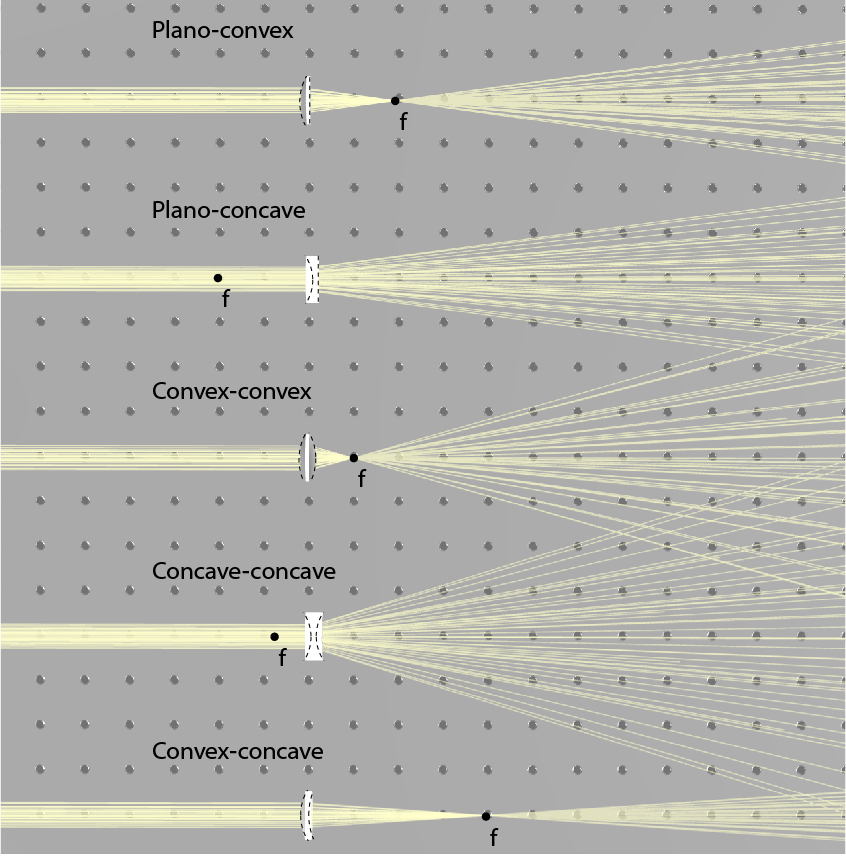Fig. 2. Illustration of five types of spherical lenses, their focal points, and their influence on collimated beams.
All the lenses presented so far are symmetric, and there is no difference between the two axes of the lens. Therefore, we can rotate them around the optical axes without impacting the beam. However, there are cylindrical lenses, where each axis can have a different curvature.

Exercise 1:

1. Identify the focal length and measure the distance from the focal point to the lens.
2. Click on the lens and enter “optical setting” then press the setting icon. Here you can change the curvature radiuses of the lens. Change the radiuses according the the table below, and run the simulations. Measure the focal length and fill in the following table:
 R1 R2 Measured f Calculated f 36 -36 40 -40 40 -1000 40 80 20 40 20 20
Note that there is a difference between the calculated focal length and the measured one due to the position of the lens. In the experiment, we measured the distance from the back surface to the focal length. This is called the back focal length (BFL). While in theory, we are calculating the distance from the focal plane to a plane inside the lens where the beams are oriented from. This distance is called the effective focal length (EFL).

### f-number and the depth of focus

The f-number of a lens is a measure for the lens’ ability to focus light. The f-number is calculated by the focal length over the diameter of the lens, as:

#### f\#=\frac fD

Where f# denotes the f-number of the lens, and D denotes the aperture of the lens. As the f-number decreases, the lens can focus light into smaller spots. The depth of focus of a lens is the length along the optical axis where the beam is focused. As the f-number of the beam increases leading to a smaller spot, the depth of focus decreases, as illustrated in Fig. 3.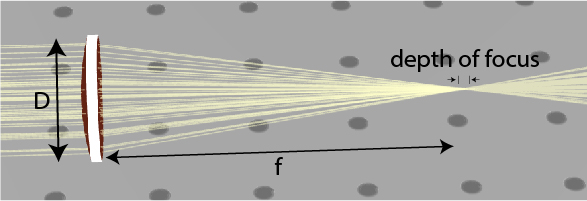Fig. 3. Illustration of a focusing lens showing the depth of focus and the parameters for evaluating the f-number of the lens.
For example, for f-number of f#=1, the focal distance equals the lens aperture, leading to a spot size of the wavelength of the beam. However, when the spot size is small, the depth of focus is also short.

### Imaging

Lenses can be utilized  to image an object. We show schematics of an imaging system with a positive lens in Fig. 4. When an object is placed at a distance u from the lens, the image is generated at a distance v, according to:

### \frac1f=\frac1u+\frac1v

A positive v indicates a real image, and a negative v indicates an imaginary image. A real image is when there is a plane in space where the light distribution is similar to the object. By placing a screen in this plane, we can observe the image. An imaginary image is when the light propagates as if there is such a plane. By observing the direction of a beam, the imaginary image can be determined, yet, an image won’t be observed on a screen that will be positioned in the in The image plane.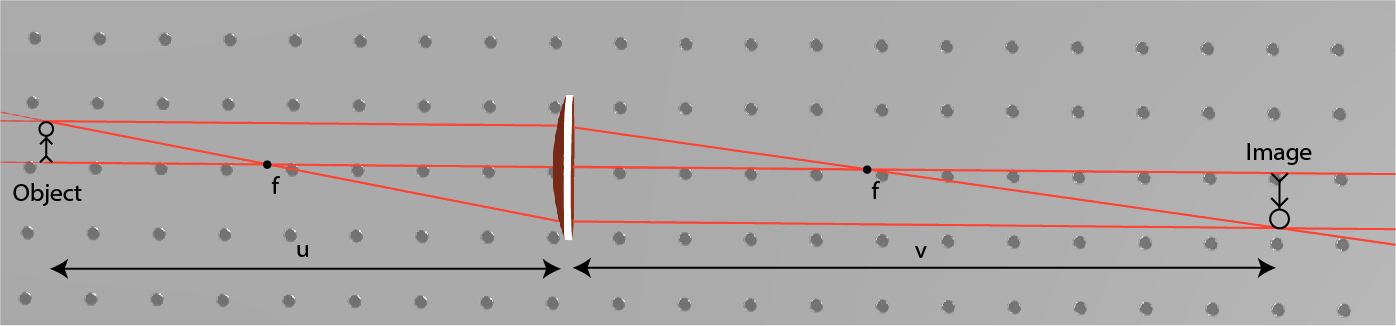Fig. 4. Imaging with a single focusing lens. When the object is placed farther from the focal distance, an actual image is generated at the other side of the lens. The image is inverted and can be either magnified or compressed.
The magnification of the image, denoted as M, is set according to

#### M=\frac vu

For a positive lens, when the object is placed farther than the focal length from the lens, the lens generates a real image according to the Imaging Equation. This is illustrated in Fig. 4. This real image can be shrinked or magnified, but it will always be inverted. If the object is farther than 2f from the lens, the image will be shrinked, but if the object is placed between f and 2f, the image will be magnified. When we move the object closer to the focal length, we increase the magnification of the image. When we place the object between the focal length and the lens, the lens generates an imaginary image. The imaginary image is not inverted, and it is magnified on the same size of the lens as the object is. Illustration van be observed in Fig. 5.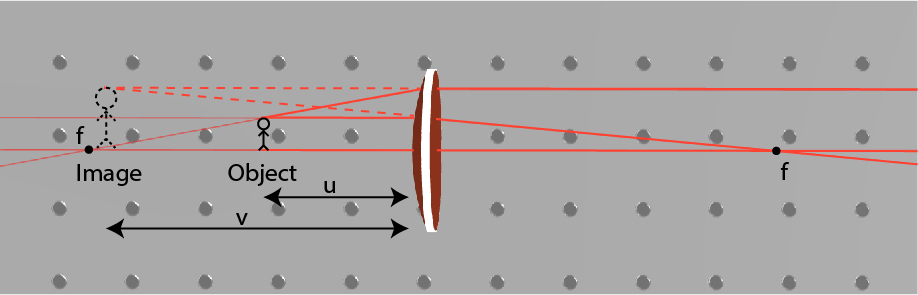Fig. 5. Imaging of an object placed between the lens and the focal distance leads to an imaginary image. The way to see the image is to look into the lens from the other side and observe the image. This is the way a magnifying glass works.

Exercise 2:

Load the file: “Lenses_ex2.opt” (press the button below to download the file). In this file, two point sources serve as the input image. The two sources are separated by 5 mm.

1. Identify the image plane. This is the plane where all the beams are focused into two spots. These two spots are the image of the two point sources.
2. Measure the distance between the sources and the lens and the distance between the image and the lens and compare them and find the focal length of the lens.
3. Measure the magnification of the image by measuring the distance between the two image-focused points. Compare this result to the calculated magnification.
4. Move the sources so the magnification of the system will be M=10.
5. Measure the magnification as a function of the distance from the lens. Fill in the following table:
 Distance from the focal length Distance from the lens Distance to the image Magnification 10 mm 5 mm 2 mm 0 mm

### Imaging with two lenses

An imaging system that is based on a single lens has limited magnification since any lens has aberrations. When trying to fabricate stronger lenses, we also increase the aberrations. One way to overcome this drawback is to resort to an imaging system with multiple lenses. Multiple lenses can lead to an imaging system with high magnification where each lens by itself is not too strong. Many imaging systems are based on multiple lenses, including microscopes and telescopes. The basic optical setup in both cases is the 4f imaging system.  The 4-f imaging system is based on two lenses situated at a distance of their combined focal lengths one from the other. This is illustrated in Fig.  6. In microscopes, we place the object next to a small lens with a short focal length and a large lens is used for imaging the object to the image plane. The magnification is the relation between the focal lengths of the two lenses.

Telescopes have a similar 4-f system, but telescopes are intended to see faint far-away objects. Therefore, the first lens is large to collect a large amount of light, and the second lens is small to make far-away objects much closer.

Exercise 3:

Load the file “Lenses_ex3.opt” with its two lenses (press the button below to download the file). One lens has a focal length of 63 mm and the other has a focal length of 630 mm.

1. Place the two lenses and the sources so the magnification will be M=10.
2. Shift one of the lenses by 1 mm. What happens to the image if the lenses are not correctly placed? Shift the other lens. Did you observe a larger or smaller change?
 Distance from the focal length Distance from the lens Distance to the image Magnification 10 mm 5 mm 2 mm 0 mm## 3DOptix worksonly on desktop!

Please go to 3doptix.com on a
desktop device, using the
Chrome or Edge browser
•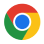•